In section-1 we learnt how to solve inequalities of the form  $$f\left( x \right) > 0\,\,{\rm{or}}\,f\left( x \right) < 0$$ , where $$f\left( x \right)$$  is a quadratic polynomial (for example, refer to example-2)

In this section, we will build on that knowledge further to solve general rational inequalities. Before proceeding, note the following fundamental facts that hold for any inequality:

(a)   If  $$a > b,\,{\rm{then}}\, - a < - b$$

(b)   If  $$a > b,\,{\rm{then}}\,ac > bc\,{\rm{only}}\,{\rm{if}}\,c > 0.$$  This means that you can multiply the two sides of an inequality by any factor only if that factor is positive. If that factor is negative, then the inequality gets reversed, i.e, if $$a > b,\,{\rm{and}}\,c < 0\,{\rm{then}}\,ac < bc.$$ Part (a) above is a special case of this fact (multiplication by –1 on both sides)

(c)   If \begin{align}a > b > 0,\,\,{\rm{then}}\frac{1}{a} < \frac{1}{b}\end{align}

(What if 0 > a > b?)

(d)   If $$\left| a \right| < b,$$ then $$- b < a < b$$ (b is positive here)

(e)   If  $$\left| a \right| > b,{\rm{then}}\,a > b\,\,{\rm{or}}\,a < - b$$

(f)  If  $${b_1} < \left| a \right| < {b_2}\,{\rm{then}}\,{b_1} < a < {b_2}\,\,{\rm{or}}\, - {b_2} < a < - {b_1}$$

(g)   If  $${a^2} < {b^2},\,\,{\rm{then}}\,\,\left| a \right| < \left| b \right|$$

Before moving on to solve inequalities, one important concept needs to be discussed. This concept is sometimes referred to as the wavy-curve method, and is used to solve general rational inequalities.

Suppose that we need to solve the following inequality:

$\frac{{\left( {x - 1} \right)\left( {x - 2} \right)}}{{\left( {x - 3} \right)\left( {x + 1} \right)}} > 0$

What we first do is multiply both sides of this inequality by $${\left( {x - 3} \right)^2}{\left( {x + 1} \right)^2}$$ {since we know for sure that this term will be positive}

This multiplication reduces the expession above to $$\left( {x - 1} \right)\left( {x - 2} \right)\left( {x - 3} \right)\left( {x + 1} \right) > 0$$

The points where the left hand side can become 0 are $$x = 1,2,3, - 1.$$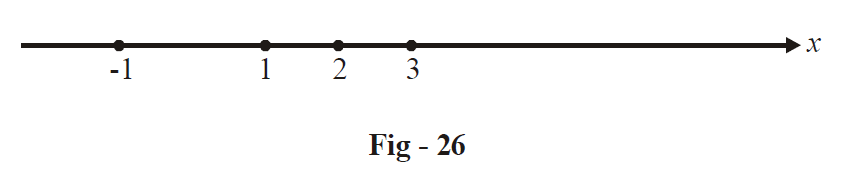If we take any value of x to the right 3, i.e x > 3, we see that all the four factors in the product above are positive, i.e for x > 3, the inequality above is satisfied.

When  $$2 < x < 3,\,\,\left( {x - 3} \right)$$  becomes negative but the other factors still remain positive, i.e the product becomes negative and the inequality is not satisfied for this interval.

Next we see that when $$1 < x < 2,$$ the product will be positive, when  $$-1 < x < {\rm{ }}1,$$  the product is negative, while it is positive again for $$x < {\rm{ }}-1.$$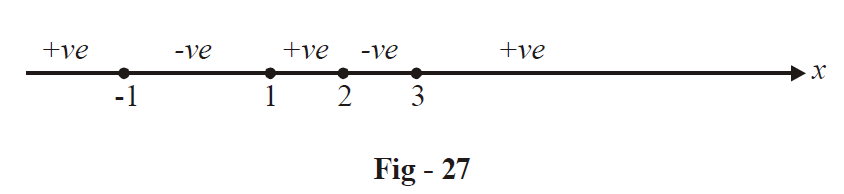Hence, the requires values of x that satisfy the given inequality are:

$x \in \left( { - \infty , - 1} \right) \cup \left( {1,2} \right) \cup \left( {3,\infty } \right)$

Generally speaking, let there be a rational function

$f\left( x \right) = \frac{{P\left( x \right)}}{{Q\left( x \right)}}$

which can be written in a factorised form as

$f\left( x \right) = \frac{{\left( {x - {a_1}} \right)\left( {x - {a_2}} \right)}}{{\left( {x - {b_1}} \right)\left( {x - {b_2}} \right)}}\,\,\frac{{.......\left( {x - {a_n}} \right)}}{{.......\left( {x - {b_m}} \right)}}$

To determine the sign of  $$f\left( x \right)$$  in different intervals, we mark the roots of both the numerator and denominator on a number line (i.e. all the  $$a_{i}^{'}s\,\text{and}\,b_{i}^{'}s$$ )

To the right of the largest root, i.e, for x > (the largest root), all the factors in both the numerator and denominator will be positive and  $$f\left( x \right) > 0.\,$$ $$f\left( x \right)\,$$  will then become negative in the preceding interval, then positive again in the next interval, and so on, i.e, $$f\left( x \right)\,$$  will alternate in sign in adjacent intervals. Now to solve  $$f\left( x \right)\, > 0,$$  we pick those values of x (those intervals) for which $$f\left( x \right)$$ is positive and to solve $$f\left( x \right) < 0,$$ we pick those intervals of x for which $$f\left( x \right)$$ is negative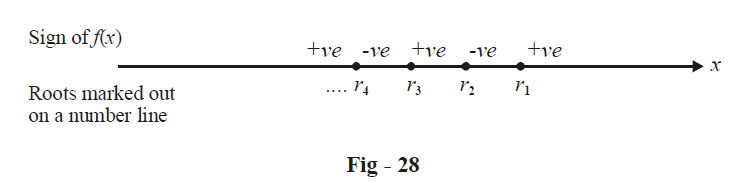Example- 26

Solve for \begin{align}&x:\frac{{2x}}{{2{x^2} + 5x + 2}} > \frac{1}{{x + 1}}\end{align}

Solution: It would be a mistake tocross-multiply and solve since $$\left( {x + 1} \right)\,{\rm{and}}\left( {2{x^2} + 5x + 2} \right)$$  are not necessarily positive. We proceed as follows:

\begin{align}&\qquad\quad\frac{{2x}}{{2{x^2} + 5x + 2}} - \frac{1}{{x + 1}} > 0\\\\ &\Rightarrow\quad \frac{{2x\left( {x + 1} \right) - \left( {2{x^2} + 5x + 2} \right)}}{{\left( {x + 1} \right)\left( {2{x^2} + 5x + 2} \right)}} > 0\\\\ &\Rightarrow\quad \frac{{ - \left( {3x + 2} \right)}}{{\left( {x + 1} \right)\left( {2x + 1} \right)\left( {x + 2} \right)}} > 0\\\\&\Rightarrow\quad \frac{{\left( {3x + 2} \right)}}{{\left( {x + 1} \right)\left( {2x + 1} \right)\left( {x + 2} \right)}} < 0\end{align}

We mark out the roots (of both the numerator and denominator) on a number line and pick the intervals where the expression is negative.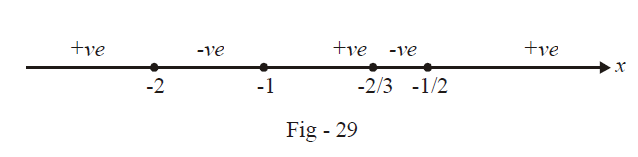The required values of x are:

$x\, \in \left( { - 2, - 1} \right) \cup \left( { - \frac{2}{3}, - \frac{1}{2}} \right)$

Example- 27

Find the values of x which satisfy \begin{align}&\frac{{8{x^2} + 16x - 51}}{{\left( {2x - 3} \right)\left( {x + 4} \right)}} < 3\end{align}

Solution: Here again, do not be tempted to cross multiply by the denominator on both sides since the denominator is not necessarily positive

\begin{align}&\qquad\qquad\frac{{8{x^2} + 16x - 51}}{{\left( {2x - 3} \right)\left( {x + 4} \right)}} - 3 < 0\\\\ &\Rightarrow \quad \frac{{\left( {8{x^2} + 16x - 51} \right) - 3\left( {2{x^2} + 5x - 12} \right)}}{{\left( {2x - 3} \right)\left( {x + 4} \right)}} < 0\\\\ &\Rightarrow\quad \frac{{2{x^2} + x - 15}}{{\left( {2x - 3} \right)\left( {x + 4} \right)}} < 0\\\\ &\Rightarrow\quad \frac{{\left( {2x - 5} \right)\left( {x + 3} \right)}}{{\left( {2x - 3} \right)\left( {x + 4} \right)}} < 0\end{align}

We now follow the same procedure as in the previous example (the alternate-interval method)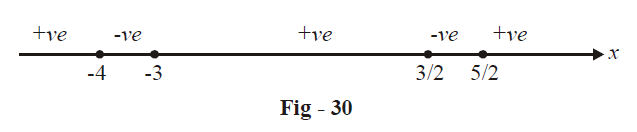The required values of x are

$x \in \left( { - 4, - 3} \right) \cup \left( {\frac{3}{2},\frac{5}{2}} \right)$

Example– 28

Solve the following inequalities for x:

(a) \begin{align}&\frac{{10x}}{{{x^2} + 9}} \le 1\end{align}            (b) \begin{align}\left| {\frac{{{x^2} - 5x + 4}}{{{x^2} - 4}}} \right| \le 1\end{align}           (c) \begin{align}\left| {\frac{{{x^2} - 1}}{{{x^2} + x + 1}}} \right| < 1\end{align}             (d)  \begin{align}{\log _{10}}\left( {{x^2} - 3x + 3} \right) \ge 0\end{align}

Solution: (a) For this case, notice that the denominator $${x^2} + 9$$ is always positive so that we can directly cross- multiply the denominator to get

\begin{align}&\qquad\quad{x^2} + 9 \ge 10x\\\\ &\Rightarrow\quad {x^2} - 10x + 9 \ge 0\\\\ &\Rightarrow\quad \left( {x - 1} \right)\left( {x - 9} \right) \ge 0\\\\ &\Rightarrow\quad x \le 1\,\,{\rm{or}}\,\,x \ge 9\end{align}

(b) From the discussion on inequalities in Section - 4, we know that this inequality is equivalent to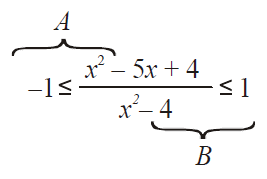We now need to consider inequalities A and B separately and then find those values of x that satisfy both these inequalities simultaneously

Inequality A:

\begin{align}&- 1 \le {{{x^2} - 5x + 4} \over {{x^2} - 4}} \quad\Rightarrow\quad {{{x^2} - 5x + 4} \over {{x^2} - 4}} + 1 \ge 0\\\\&\qquad\qquad\qquad\qquad\;\quad\Rightarrow\quad {{2{x^2} - 5x} \over {{x^2} - 4}} \ge 0\\\\&\qquad\qquad\qquad\qquad\;\quad\Rightarrow\quad {{x\left( {2x - 5} \right)} \over {\left( {x - 2} \right)\left( {x + 2} \right)}} \ge 0\end{align}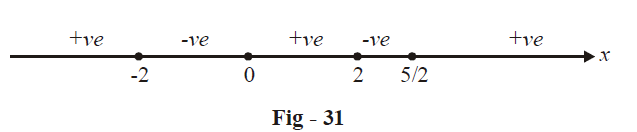$\Rightarrow x < - 2\,\,{\text{or}}\,\,0 \leqslant x < 2\,\,\,{\text{or}}\,\,\,x \geqslant \frac{5}{2}$

(Note above that x cannot take the values –2 or 2 since the denominator would then become 0).

Inequality B:

\begin{align}&\frac{{{x^2} - 5x + 4}}{{{x^2} - 4}} \le 1 \quad\Rightarrow\quad \frac{{{x^2} - 5x + 4}}{{{x^2} - 4}} - 1 \le 0\\\\ &\qquad\qquad\qquad\quad\;\quad\Rightarrow\quad \frac{{8 - 5x}}{{{x^2} - 4}} \le 0\\\\ &\qquad\qquad\qquad\quad\;\quad\Rightarrow\quad \frac{{5x - 8}}{{\left( {x + 2} \right)\left( {x - 2} \right)}} \ge 0\end{align}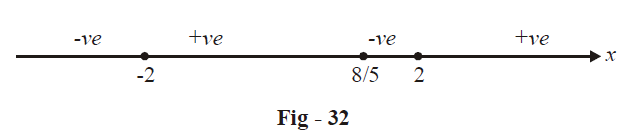$\Rightarrow - 2 < x \le \frac{8}{5}\,\,\,{\rm{or}}\,\,x > 2$

We now find the intersection of the solutions to these two inequalities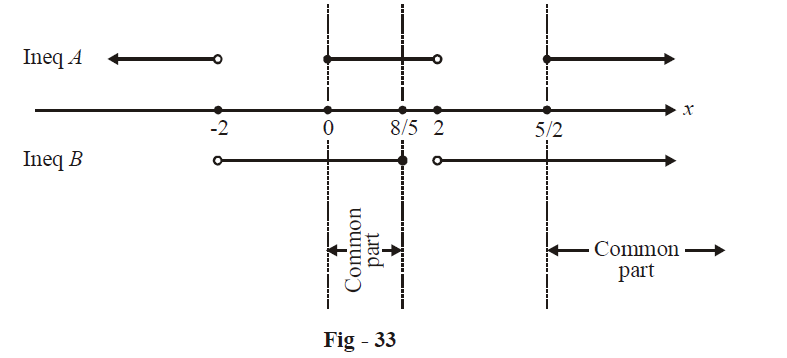The solution for x is therefore:

$x \in \left[ {0,\frac{8}{5}} \right]\, \cup \left[ {\frac{5}{2},\infty )} \right.$

(c)  This inequality can be written equivalently as

$- 1 < \frac{{{x^2} - 1}}{{{x^2} + x + 1}} < 1$

Notice (or recall: example-1 Part C) that the denominator of the given rational expression is always positive and hence it can directly be cross-multiplied across both the inequalities to get

$- {x^2} - x - 1 < {x^2} - 1 < {x^2} + x + 1$

The two inequalities considered separately are

\begin{align}&\;\;\;\;\;\;{x^2} - 1 < {x^2} + x + 1 \quad \Rightarrow&\;\; x > 2\\\\ &- {x^2} - x - 1 < {x^2} - 1 \quad\Rightarrow&\quad 2{x^2} + x > 0\\\\ &\qquad\qquad\qquad\qquad\qquad\;\;\;\Rightarrow& x\left( {2x + 1} \right) > 0\\\\ &\qquad\qquad\qquad\qquad\qquad\;\;\;\Rightarrow& x < - 1/2\,\,{\rm{or}}\,\,x > 0\end{align}

The common part of the two solutions can be easily seen to be

$x \in \left( { - 2, - \frac{1}{2}} \right) \cup \left( {0,\infty } \right)$

(d) Since $${\log _{10}}\left( {{x^2} - 3x + 3} \right) \ge 0$$

\begin{align}&\Rightarrow \quad {x^2} - 3x + 3 \ge {10^0} = 10\\\\ &\Rightarrow\quad {x^2} - 3x + 2 \ge 0\\\\ &\Rightarrow\quad \left( {x - 1} \right)\left( {x - 2} \right) \ge 0\\\\ &\Rightarrow\quad x \le 1\,\,\,\,\,{\rm{or}}\,\,\,\,\,x \ge 2\end{align}

TRY YOURSELF - IV

1. Solve for x:  \begin{align}\frac{4}{{1 + x}} + \frac{2}{{1 - x}} < 1\end{align}

2.  Solve for x :  \begin{align}\frac{{\left( {x - 1} \right)\left( {x - 2} \right)}}{{x + 5}} > 1\end{align}

3.  Solve for x :  \begin{align}\left| {\,\frac{{{x^2} - 3x - 1}}{{{x^2} + x + 1}}\;} \right| < 3\end{align}

4.  Solve for x : \begin{align}\frac{{|x - 3|}}{{{x^2} - 5x + 6}} \ge 2\end{align}

5.  Solve the following inequalities for x:

 (a) $${\log _3}\left( {2{x^2} + 6x - 5} \right) > 1$$ (b) $${\log _{10}}\left( {{x^2} - 2x - 2} \right) \le 0$$ (c) $${\log _{x - 2}}\left( {3{x^2} - x - 1} \right) > 0$$ (d) $${\log _x}\left( {2x - \frac{3}{4}} \right) > 2$$ (e) $${\log _{{x^2} - 1}}\left( {x + 1} \right) < 1$$ (f) $${\log _{9{x^2}}}\left( {6 + 2x - {x^2}} \right) \le 0.5$$ (g) $${\log _x}2 \cdot {\log _{2x}}2 \cdot {\log _2}4x > 1$$ (h) \begin{align}{\log _2}\left( {\frac{{x - 4}}{{2x + 5}}} \right) < 1\end{align}

Learn from the best math teachers and top your exams

• Live one on one classroom and doubt clearing
• Practice worksheets in and after class for conceptual clarity
• Personalized curriculum to keep up with school